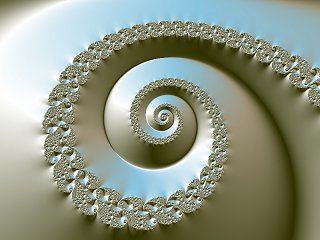Search IntMath
Close

# Spiral Fractal

By Murray Bourne, 13 Nov 2004Hey - I love these things! Here's one called "Spiral Fractal".

Is this just a 'nerd' thing or does anyone else find them attractive?

Fractals are built on specific iterations of complex numbers. You can see some mathematical background on the concept here: Fractals, in the Complex Numbers chapter of IntMath.

Image credit: This and other fractal images are freely available from here.

### Comment Preview

HTML: You can use simple tags like <b>, <a href="...">, etc.

To enter math, you can can either:

1. Use simple calculator-like input in the following format (surround your math in backticks, or qq on tablet or phone):
a^2 = sqrt(b^2 + c^2)
(See more on ASCIIMath syntax); or
2. Use simple LaTeX in the following format. Surround your math with $$ and $$.
$$\int g dx = \sqrt{\frac{a}{b}}$$
(This is standard simple LaTeX.)

NOTE: You can mix both types of math entry in your comment.